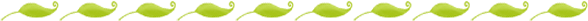The Development of Mathematical RepresentationAccording to the research of David Sousa, children progress through three stages of mathematical understanding as they develop an understanding of concepts. The stages are Concrete, Representational (Pictorial), and Abstract. It will be important to remember that each of our children will be in a different stage of development for each concept that we are teaching, and, therefore, it is important to differentiate the method by which the children are allowed to work with problems. Differentiating in this way is sometimes known as the CRA (or CPA) approach. First, let's define the different stages of development:

Concrete

All children must start here when learning mathematical concepts. Concrete models tie mathematics to the real world and include anything that the child can use physically to represent a problem.

Representational/Pictorial

The representational stage provides the mental scaffolding for children to move their mathematical understanding from concrete to abstract. In this stage, children are able to use visual or pictorial representations to represent concrete examples. Teachers deliberately help children see how pictorial representations tie to concrete examples.

Abstract

The abstract level of thinking represents mathematical thinking symbolically. It is important to realize that this is the final level of understanding for children, and that we must help each child through the first two stages before they will be able to grapple with abstract representations. "Numerals were developed to signify the meaning of counting. Operational symbols like + and - were constructed to represent the actions of combining and comparing. While these symbols were initially developed to represent mathematical ideas, they become tools, mental images, to think with. To speak of mathematics as at mathematizing demands that we address mathematical models and their developments. To mathematize, one sees, organizes, and interprets the world through and with mathematical models. Like language, these models often begin as simply representations of situations, or problems, by learners... These models of situations eventually become generalized as learners explore connections between and across them" (Fosnot and Dolk, 2001)

.

Many lessons in kindergarten can be specifically designed to move children from one stage of understanding to another. For example, one lesson could ask groups of children to count out objects from a bag and then draw a picture of the objects they found (Concrete to Pictorial). The teacher could then write the number each group found in their bags on the board (Pictorial to Abstract). Lessons can also be designed where each stage of development can be used to answer the question.

Here is such a lesson: The teacher places a container in view of the children and tells them their are five bears inside. Some of the bears are red and some are blue. How many of each color could be inside? Children can use their own sets of bears to answer the question. They could draw a picture. They could use numbers and equations. The important part of the lesson is that each child is developing an understanding of the part/whole relationships of the number 5, and allowing each child to work within his own stage of understanding will better strengthen his mathematical knowledge.